# Basics of Hydraulic Surge or Water Hammer

What is hydraulic surge or water hammer? How it develops, its impact all these information is covered in this post as Basics of Hydraulic Surge or Water Hammer.

## Introduction

Inertia is the tendency of matter to remain in its existing state of motion, unless acted upon by outside forces. More energy is required to change steady state motion than to maintain it.

Thus, more energy is expended to increase or decrease flowing velocity in a pipeline than is necessary to maintain the liquid’s steady state motion. One measure of the energy used to change liquid motion is pressure. If velocity is changed quickly by the application of much energy in a short period of time, the pressure change will be more significant than when the same amount of energy is expended over a long time period. Thus, the energy applied to change pressure by 100 psi in one second is the same amount of energy as that applied for 10 seconds to change pressure by 100psi.

## What is hydraulic surge?

Any change from steady state conditions creates a temporary variation in pressure or flow called hydraulic / pressure transients. Or in other words they occur when the flow of fluid in a pipeline is abruptly changed. Hydraulic transients are commonly called hydraulic surge, hydraulic shock, or water hammer.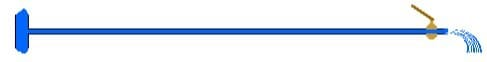Hydraulic Shock Valve Closing Pressure surges are produced by unsteady flow resulting from either acceleration or deceleration of a liquid. Every flowing fluid accumulates kinetic energy (velocity head), the strength of which is determined by the weight of the fluid and the speed with which it is moving. If the speed of this fluid is changed, i.e., suddenly slowed or stopped, the kinetic energy is transformed into pressure energy and causes the normal system pressure to increase.

This unsteady state phenomenon deals with the change between kinetic energy and pressure energy, which may be positive or negative. If the pressure induced exceeds the pressure rating of a pipe given by the manufacturer, the pipe may rupture. Detrimental consequence may result unless a pressure protection device is installed. The hydraulic engineer, water supply designer and pumping system operator have the responsibility to ensure the surges due to water hammer are within acceptable limits and can be dampened as quickly as possible. These conditions can result in piping failure, damage to pumps, fittings, instrumentation and other system components.

These hydraulic/ pressure transients, or surges, can be created in piping systems due to the following reasons.

• Sudden opening or closing of valves in a pipeline (quick acting valves)
• Rapid starting or stopping of the pumps in a pumping system
• Sudden increases in the inflow to a river or a sewer due to flash storm runoff
• Operating errors or malfunctioning of equipment.

Their magnitude is a function of the

• Change in flowing velocity;
• Liquid density;
• Sound speed in the liquid and piping system.

These hydraulic transients may range in importance from a slight pressure and/or velocity change, to sufficiently high vacuum or pressure to collapse or burst pipes and fittings or damage pumps.

Surge (often called Water hammer) causes many of the problems experienced in liquid systems: high and low pressures; severe pipe loads; unwanted trips and shutdowns; pipe movement and vibration; bursting disc rupture; and equipment damage.

When a fluid is flowing with a constant velocity through a pipe and a downstream valve is closed, the following sequence of events takes place:

• The water adjacent to the valve is stopped; the momentum in the fluid is converted to a pressure head, which compresses the fluid and expands the pipe walls.
• The flow of adjacent particles within the water is successively brought to rest, transforming momentum into pressure energy.
• When the water is compressed due to the sudden stop., the pressure wave travels upstream to the end of the pipe at wave velocity – “A”. If the pipe length is “L”, then after L÷A seconds the wave will be at the upstream end. The pressure wave, after reaching the upstream end (i.e. valve, etc.) of the pipe, is reversed and returns to the downstream valve at 2L÷A seconds after valve closure.
• During this time, the fluid pressure and the pipe stress will be greater than normal. If the fluid were ideal, there would be no resistance to flow and surges would continue to repeat indefinitely. With friction and pipe elasticity, the pressure amplitudes diminish gradually until original momentum is absorbed.
• The explanation of water hammer in the previous paragraphs assumes instantaneous closure. But valves require a finite time to close. If closure takes place in a time less than 2L÷A, then it is termed “critical” (TC) and the incremental pressure rise is shown in the table. The table shows the increase in water pressure of a system for each one foot per second flow rate and each 100 feet of PVC pipe. For other “Run” lengths and “Velocities” multiply “Peak Pressure Surge” accordingly.

V     =    Velocity Change, (Feet/Second)   =        1

L      =    Pipe Run Length, Ft.                      =        100

W    =     Fluid Density, #/Ft.3                      =        62.4 (Water)

K     =     Modulus Of Fluid, Psi.                  =        300,000 (Water)

Dr    =     Dimension Ratio (In./In.)             =        Pipe O.D.÷ Wall Thickness

Tc    =     Critical Valve Close Time (Sec.)  =        (2 X L)÷A

P      =     Pressure Change (Psi) (Peak)      =        (W X A X V)÷4,636.6, (Adds To Flow Press.)

A      =     Wave Velocity (Feet/Second)      =        4,660÷[1+{K X (-2+Dr)÷E}]½

E      =     Modulus Of Material Psi.             =        400,000 ( Pvc)

 Dimension (DR)   Ratio Rated Pressure (A) Wave Velocity (Tc) Critical Close Time (P) Peak Surge Pressure All Sizes 13.5 315 1,502 0.13 20.21 All Sizes 17 250 1,331 0.15 17.92 All Sizes 21 200 1,193 0.17 16.06 All Sizes 26 160 1,069 0.19 14.39 All Sizes 32.5 125 954 0.21 12.84 Schedule 40 pipe ½ 7.7 600 2,029 0.10 27.31 ¾ 9.3 480 1,831 0.11 24.65 1 9.9 450 1,771 0.11 23.83 1¼ 11.9 370 1,605 0.12 21.61 1½ 13.1 330 1,526 0.13 20.54 2 15.4 280 1,402 0.14 18.87 2½ 14.2 300 1,463 0.14 19.69 3 16.2 260 1,365 0.15 18.37 4 19 220 1,257 0.16 16.91 5 21.6 190 1,176 0.17 15.83 6 23.7 180 1,121 0.18 15.09 8 26.8 160 1,053 0.19 14.17 Schedule 80 pipe ½ 5.7 850 2,398 0.08 32.28 ¾ 6.8 690 2,173 0.09 29.24 1 7.4 630 2,074 0.10 27.91 1¼ 8.7 520 1,898 0.11 25.55 1½ 9.5 470 1,810 0.11 24.37 2 10.9 400 1,682 0.12 22.64 2½ 10.4 420 1,725 0.12 23.21 3 11.7 370 1,620 0.12 21.8 4 13.4 320 1,508 0.13 20.29 5 14.8 290 1,431 0.14 19.26 6 15.3 280 1,407 0.14 18.93 8 17.3 250 1,319 0.15 17.76
• Peak surge pressure (P) is directly proportional to the velocity changes (V). P tabled above is per foot/sec. Velocity change.
• Critical valve close time (TC) is directly proportional to the pipe run length (L). TC tabled above is per 100 ft. Of run length.
• If actual valve close time (TA) is less than the critical time (TC), then the peak surge is as shown, but if TA is greater than TC, then the approximate pressure surge is (PA) = (0.027 x L x V)÷TA (psi).
• Air slugs in a system can create surge velocities far in excess of the system design flow rate. Abrupt flow changes and high flow rates can compound to create very high hydraulic pressure shocks.

## Study of Hydraulic Transients

The study of hydraulic transient began with the investigation of sound wave propagation in air, followed by theories derived by Joukowski (1900; a Russian scientist) and Allievi (1903; a French researcher). In 1897, Joukowski conducted extensive experiments in Moscow on pipes with diameters ranging from 50mm to 150mm and lengths from 350m to 7620m. He developed a formula for the surge wave velocity, the rise of pressure and velocity reduction. He also discussed the effect of various closing rates (gradual or instantaneous) of a valve.

Joukowski’s Law: water hammer head H at instantaneous valve closure in a rigid pipe

Maximum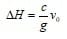(1)

where

vo = flow velocity before valve closure

c = wave celerity or velocity of sound in liquid

If the pressure in a closed conduit drops below the vapour pressure of a liquid, then cavities (where the water column is separated by an air space) are formed. This phenomenon is called water column separation.. This is the worst condition for water hammer, and may result in the pipeline not being able to withstand the external forces and hence collapse. This can be a problem for large diameter thin walled pipes and plastic pipes with low pressure rating or carrying hot liquids. The rejoining of separated water columns can produced high pressures putting the pipeline at risk. The frequent expansion and contraction may cause failure of cement lining in metal pipes.

For acceleration and deceleration of an incompressible liquid in a pipe, a head loss term due to unsteady motion of changing velocity “dv” in time “dt”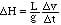(2)

is included in the Bernoulli equation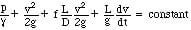(3)

## Pressure Waves Due To Instantaneous Velocity Change

### Wave propagation and reflection in a single rigid pipe

In Physics we learned that the frequency (f) of a sound wave in an pipe with one end closed is f = l/c = 4L/c, where L is the length of the pipe, l is wavelength, and c is the velocity of sound wave in a liquid. If the closure time t <= 2L/c, it is called sudden or instantaneous closure; and gradual closure if t 4L/c.

Let’s now consider the fluctuations of the excess pressures caused by an instantaneous closure of a valve located at the downstream end of a pipe. If the frictional loss along the pipe is neglected, the sudden change in fluid velocity from v = vo to v = 0 at the valve generates a surge wave propagating upstream, accompanying by a positive excess pressure head D H. The surge wave eventually reflects from the reservoir and advancing downstream. On reaching at the closed valve, it then propagates upstream with a negative surge wave, and so on. The fluctuations in pressure head D H throughout the first cycle are depicted in the following sketches. In reality, the magnitude of the excess pressure dampens due to friction.

The increase in water head is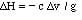(4)

and celerity of water hammer waves in a compressible fluid in a rigid pipe is given by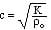where the bulk modulus of elasticity K is defined as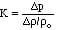(5)

### Celerity of transient in a non-rigid pipe

In addition to the bulk modulus of elasticity K of the fluid, the velocity of water hammer waves depends also on the elastic properties of the conduit and external restraints.

Neglecting the presence of free gases, Halliwell (1963) provided a general expression: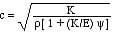(6)

where

E = Young’s modulus of elasticity of the conduit walls

y = a non-dimensional parameter depends on the elastic properties of the conduit

which is a function of (e, wall thickness; D, conduit diameter and Poisson ratio n)

• Rigid conduits y = 0 (7)
• Thin-walled elastic conduits
1. conduit anchored against longitudinal movement throughout its length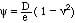(8.a)
2. conduit anchored against longitudinal movement at the upper end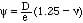(8.b)
3. conduit with frequent expansion joints (8.c)
• Polyvinyl Chloride (PVC) and reinforced plastic pipe
• Equation (6) can be used provided proper values of K and E are used.
• Thick-walled elastic conduits
• Non-circular conduits
• Tunnels through solid rock

Some common values of E, n, K:

 Material E (GPa) Poisson’s v K (GPa) Density (kg/m3) Brass 78-110 0.36 Mild steel 200-212 0.27 Plastic – Perspex 6.0 0.33 – Polyethylene 0.8 0.46 – PVC rigid 2.4-2.75 Water, fresh 2.19 999 (20C) Water, sea 2.27 1025 (15C)

### Partial valve closure

If the response of water hammer is linearly elastic, when a valve is partially closed which reduces the velocity by “dv” from vo, then from equation (4)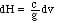(9)

and it can be shown that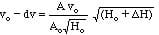(10)

where
Ho = pressure head at valve with the valve fully open

Ao = normal valve orifice area when fully open

A = valve orifice area after partial closure

If partial closure is sudden or instantaneous, Delta H in equation (10) is given by equation (9); if gradual, Delta H is given by equation (2), ie. Delta H = (L/g) (dv/dt).

When the valve is closed stepwise in time T and the rate of valve closure dA/dt is uniform, then A/Ao = 1 – dt/T (11)

From equations (10) and (11),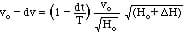(12)

In equation (12), “dt” is the time in which the pipe velocity is reduced by “dv” and “dt/T” may be taken as a conveniently small fraction (Smith 1960).

• Example of partial valve closure

<Q A valve at the end of a pipeline of length L = 750 m is closed in 10 equal steps each of 2L/c seconds where c = 1200 m/s. The initial head at the valve is 120 m and initial flow velocity is 3.5 m/s. Determine the pressure head at the valve after 1.25 and 2.5 seconds and compare with the result for a sudden closure.

<A From equations (9) for Delta H and (12), Delta v can be calculated,

where each step Delta t = 2L/c = 2 x 750/1200 = 1.25 sec; Ho = 120 m, vo = 3.5 m/s

1) after t = 1 Delta t = 1.25 s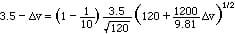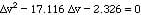Therefore Delta v = 0.137 m/s

and H (at t = 1.25 s) = Ho + Delta H = 120 + (1200/9.81) x 0.137 = 136.76 m

2) after t = 2 Delta t = 2.5 s

new vo = 3.5 – 0.137 = 3.363 m/s, new Ho = 136.76 m; entering equation      (15)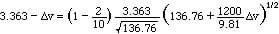Therefore Delta v = 0.316 m/s

and H (at t = 2.5 s) = Ho + Delta H = 136.6 + (1200/9.81) x 0.316 = 175.4 m

and so on with new t = 3Delta t, … 10Delta t

However, if the valve is closed suddenly, then Delta H = (c/g) vo = 428 m theoretically.